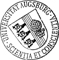## Growth Rates for Semiflows on Hausdorff Spaces

• In this paper, we present a theory of vector-valued growth rates for discrete- and continuous-time semiflows on Hausdorff spaces. For a given compact flow-invariant set M and an associated growth rate, we introduce the uniform growth spectrum over M, and associated real-valued spectra via projections of the vector-valued spectrum onto one-dimensional subspaces. We show that these real-valued spectra are closed intervals if M is additionally connected. We also define the Morse spectrum associated with a growth rate by evaluating the growth rate along chains. Moreover, we relate the uniform growth spectrum to the Morse spectrum and we analyze the meaning of limit sets for the long-time behavior of growth rates.

• Dokument_1.pdfAuthor: Christoph KawanGND, Torben Stender urn:nbn:de:bvb:384-opus4-12302 https://opus.bibliothek.uni-augsburg.de/opus4/1535 Preprints des Instituts für Mathematik der Universität Augsburg (2011-09) Preprint English Universität Augsburg 2011/06/06 Halbflüsse; Morse-Spektrumsemiflows; growth rates; Lyapunov exponents; Morse spectrum Ljapunov-Exponent; Wachstumsrate; Dynamisches System; Hausdorff-Raum Mathematisch-Naturwissenschaftlich-Technische Fakultät Mathematisch-Naturwissenschaftlich-Technische Fakultät / Institut für Mathematik 5 Naturwissenschaften und Mathematik / 51 Mathematik / 510 MathematikDeutsches Urheberrecht